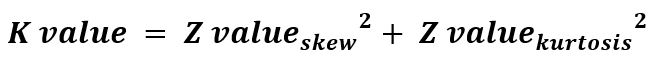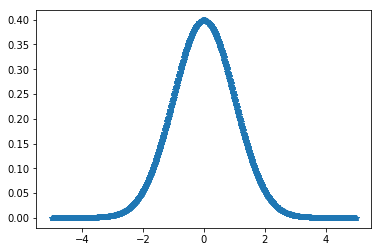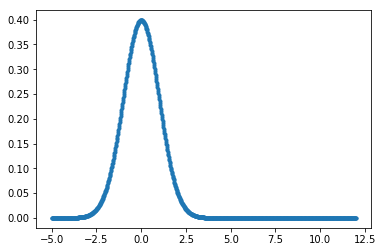# scipy stats.normaltest() function | Python

• Last Updated : 11 Feb, 2019

`scipy.stats.normaltest(array, axis=0)` function test whether the sample is different from the normal distribution. This function tests the null hypothesis of the population that the sample was drawn from.

Parameters :
array : Input array or object having the elements.
axis : Axis along which the normal distribution test is to be computed. By default axis = 0.

Returns : k2 value and P-value for the hypothesis test on data set.Code #1:

 `# Performing normaltest``from` `scipy.stats ``import` `normaltest``import` `numpy as np ``import` `pylab as p `` ` `x1 ``=` `np.linspace( ``-``5``, ``5``, ``1000` `)``y1 ``=` `1.``/``(np.sqrt(``2.``*``np.pi)) ``*` `np.exp( ``-``.``5``*``(x1)``*``*``2`  `)`` ` `p.plot(x1, y1, ``'.'``)`` ` `print``( ``'\nNormal test for given data :\n'``, normaltest(y1))`

Output :

```Normal test for given data :
NormaltestResult(statistic=146.08066794511544, pvalue=1.901016994532079e-32)
```

Code #2:

 `# Performing normaltest``from` `scipy.stats ``import` `normaltest``import` `numpy as np ``import` `pylab as p `` ` `x1 ``=` `np.linspace( ``-``5``, ``12``, ``1000` `)``y1 ``=` `1.``/``(np.sqrt(``2.``*``np.pi)) ``*` `np.exp( ``-``.``5``*``(x1)``*``*``2`  `)`` ` `p.plot(x1, y1, ``'.'``)`` ` `print``( ``'\nNormal test for given data :\n'``, normaltest(y1))`

Output :

```Normal test for given data :
NormaltestResult(statistic=344.05533061429884, pvalue=1.9468577593501764e-75)
```

My Personal Notes arrow_drop_up